# Division property of equalityJane has two cakes of equal sizes. She has 8 guests at the party. She decides to divide both the cakes evenly throughout. How will she be able to do this?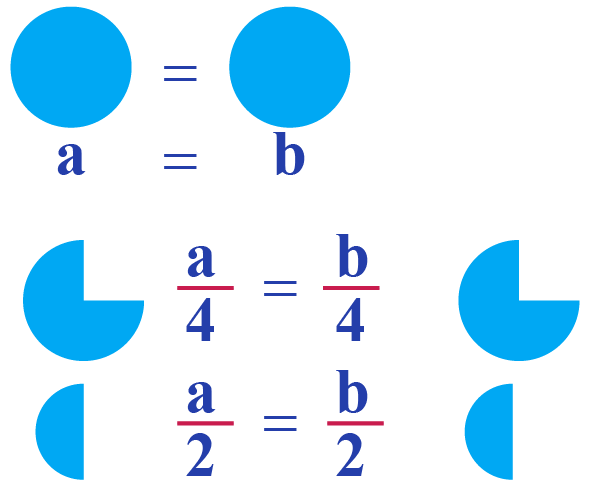Thus dividing both the cakes evenly to get the balance while using them is the division property of equality. Let's learn more about it in detail.

In this mini lesson let us learn about the division property of equality formula, division property of equality in geometry, division property of equality with fractions, division property of equality proof, division property of equality calculator and division property of equality angles.

### What Is Division Property of Equality?

If $$a$$, $$b$$, $$c$$ are the real numbers, such that, then the division property of equality formula is given by:

 If $$a = b$$ and $$c \neq 0$$, then $$\dfrac{a}{c} = \dfrac{b}{c}$$

Consider the equation $$18 = 18$$

Divide both sides by 2

\begin{align}\dfrac{18}{2} &= \dfrac{18}{2}\\ 9 &= 9\\\text{LHS} &= \text{RHS}\end{align}

The equation still remains balanced.

### Definition

Division property states that when we divide one side of an equation by a number, we should divide the other side of the equation by the same number so that the equation remains balanced.

### Division Property of Equality Proof

The following picture illustrates the division property of equality in Algebra in solving linear equations.

If $$5x = 25$$, then $$x = 5$$ on dividing by 5 on both sides.

Thus, we apply the division property of equality formula here to solve for $$x$$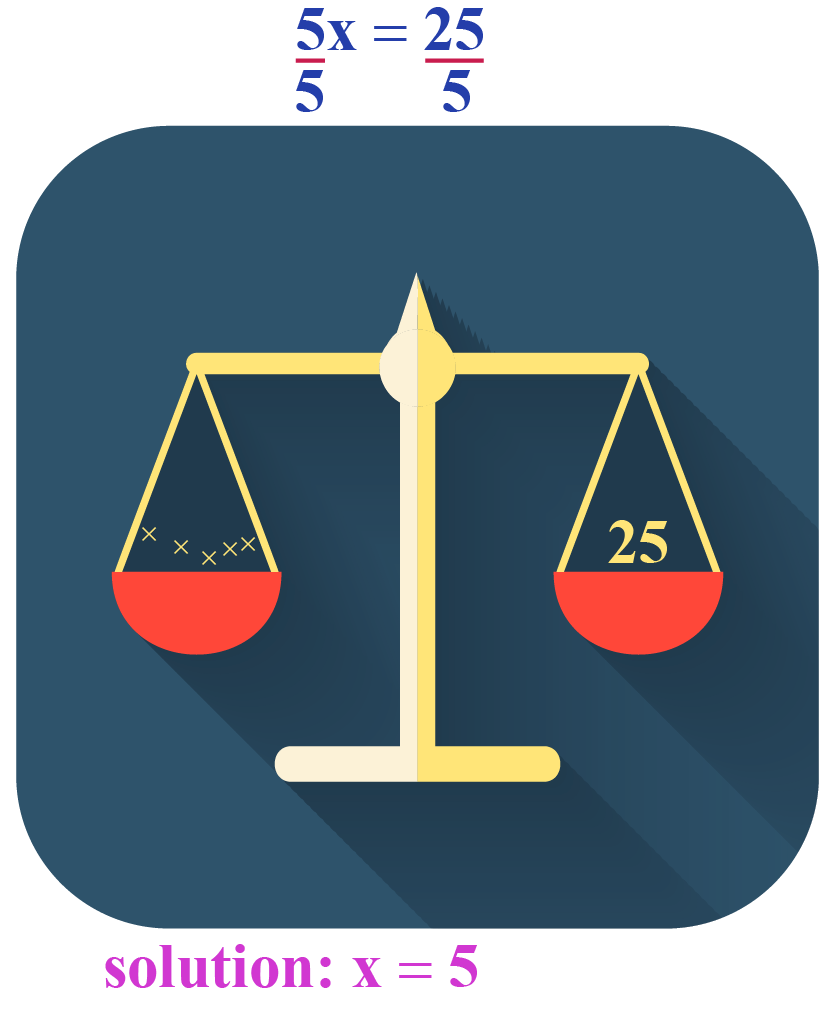You can verify if $$x = 5$$ is the solution of the given equation by substituting $$x = 5$$ in the equation.

\begin{align}\text{LHS} &=5 x\\ &= 5\times x\\ &= 5\times 5\\ &= 25\\ &= \text{RHS}\end{align}

Thus we obtain the division property of equality proof.

### Formula of Division Property of Equality

The formula of division property of equality is given as:

 If $$a = b$$ and $$c = d$$, then $$\dfrac{a}{c}= \dfrac{b}{d}$$

Example

1. To find the value of the unknown variable, we use this division property along with the other properties of equality.

Consider solving this linear equation, $$3x - 2 = 7$$

\begin{align*}3x - 2 + 2 &= 7 + 2 \text{ (addition property)}\\\\ 3x &= 9\\\\\dfrac{3x}{3} &= \dfrac{9}{3}\,\text{(division proerty})\\\\x &= 3\end{align*}

2. Consider equation $$2x = 1$$

To solve for $$x$$, divide both sides by 2.

\begin{align}\dfrac{2x}{2} &= \dfrac{1}{2}\,\text{(division property)}\\\\ x &= \dfrac{1}{2}\end{align}

### Division Property of Equality Calculator

In the following simulation, enter the equation, try to solve the equations and check your answers with the step-by-step procedure in solving the equation.• When the equations to be solved are in the form of multiplication, we need to use the division property of equality. $$10 x = 100$$, here is the hint for us to use the division property. Divide both sides by 10.

### Division Property of Equality in Geometry

Congruent angles have equal measures.

1. Division Property of Equality in Geometry is used along with the properties of congruence.

Given are two congruent angles. We need to find the measures of the angles.

$$5x - 8$$ and $$3x + 4$$ are the two congruent angles.

$$\therefore 5x-8 = 3x +4$$

We need to solve for $$x$$ and then the angles by using the division property of equality angles.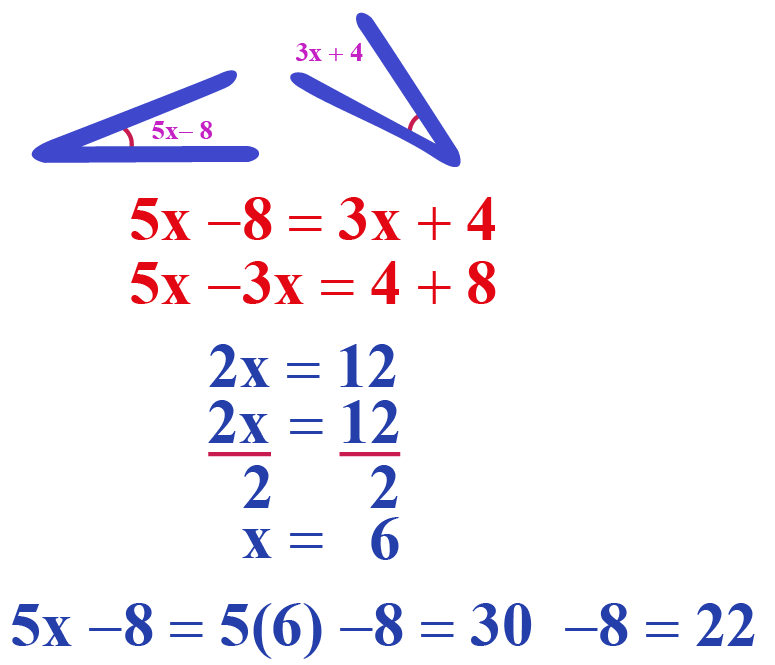Thus we find that both the angles are equal to $$22^\circ$$

2. Division Property of Equality Angles is used to find the unknown angles.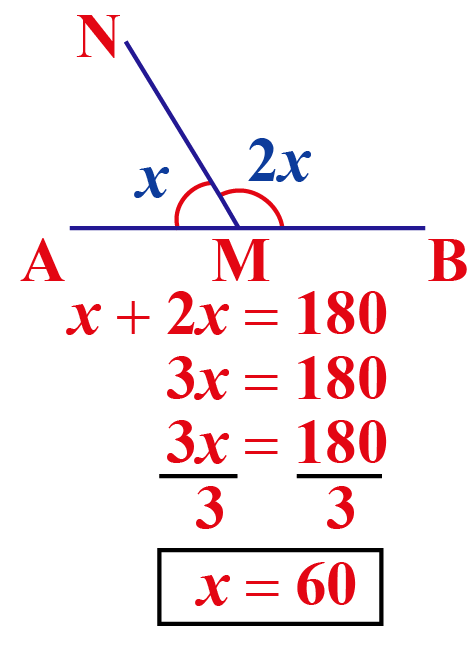$$\angle AMN + \angle BMN = 180^\circ$$ $$\because$$ the angles form a linear pair and are supplementary.

\begin{align}x + 2x &= 180\\\\3x &= 180 (\text{division property)}\\\\ x &= 60\end{align}

### Division Property of Equality with Fractions

\begin{align}10\text{v} &= 2\\\\\dfrac{10 \text{ v}}{10} &= \dfrac{2}{10}\text{(divide both sides by 10)}\\\\\text{v} &= \dfrac{1}{5}\end{align}

You can verify if $$\text{ v} = \dfrac{1}{5}$$ by substituting in the equation.

\begin{align}\text{LHS} &= 10 \text{v}\\\\ &= 10\times \dfrac{1}{5}\\ &= 2\\\\ &=\text{RHS} \end{align}

Thus verified.

### What Are the 8 Properties of Equality?

The following are the 8 properties of equality:

PropertyApplication
SubstitutionIf $$a = b$$, then "b" can replace "a" in any expression.
AdditionIf $$a = b$$, then $$a + c = b + c$$
SubtractionIf $$a = b$$, then $$a - c = b - c$$
MultiplicationIf $$a = b$$, then $$ac = bc$$
DivisionIf $$a = b$$and  $$c \neq 0$$, then $$\dfrac{a}{c}= \dfrac{b}{c}$$
Symmetricif $$a= b$$, then $$b = a$$
Reflex$$a = a$$ i.e. the number is equal to itself
TransitiveIf $$a = b$$ and $$b = c$$ , then $$a = c$$Important Notes

• Division property of equality along with the other properties allows us to solve the equations.
• Remember to divide by the same number on both sides of the equation to balance the equation.

The girls went to the canteen and bought three muffins and two coffees that together cost $17. If the cost of one coffee is$4, What is the cost of 1 muffin?Solution

First, let us convert the given statement into an equation.

Let $$a$$ be the cost of 1 muffin and b be the cost of 1 coffee.

Then we have $$3a + 2b = 17$$

Given that $$b = 4$$, then we have 2 coffees for 8 Thus we have, \begin{align}3a + 8 &= 17\\\\ 3a + 8 - 8 &\!=\! 17 - 8\text{(subtraction property)}\\\\3 a &= 9\\\\\dfrac{3a}{3} &= \dfrac{9}{3}\text{(division property)}\\\\a&=3\end{align}  $$\therefore$$ The cost of a muffin is3

Andrew is about to make a recipe that requires equal amount of flour and sugar.

He knows the flour measures 144 pounds. His sugar pack after the last usage has been marked as $$12 (x+4)$$. He places the packs of flour and sugar on the pans. They get balanced. What could be the value of $$x$$?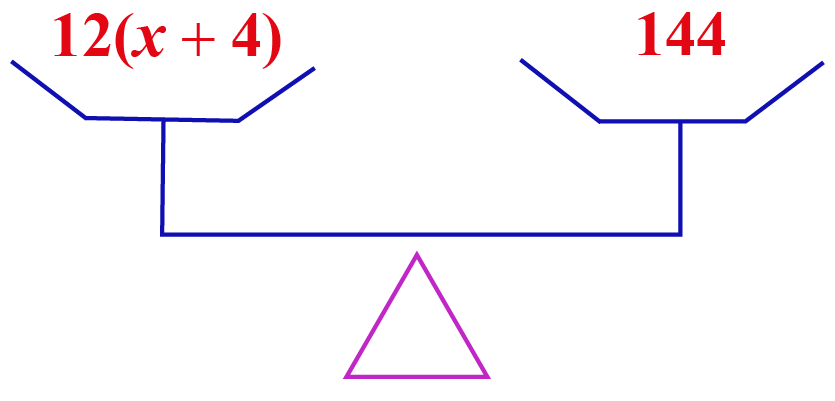Solution

Since the pans get balanced, the quantities of sugar and flour should be equal.

i.e.  $$12 (x+4) = 144$$

We find that this involves multiplication and hence division property could be used.

\begin{align}\dfrac{12 (x+4)}{12} &= \dfrac{144}{12}\\\\ x+4 &= 12\\\\\text{By subtraction property,}\\ x+ 4 -4 &= 12 - 4 \\\\ x &= 8\end{align}

a) The amount earned by Shawn per hour is $25. He has earned$100. How many hours did he work?

b) Shawn completes one-eighth of his work in 4 hours. How much of work will he complete in an hour?

Solution

Let us convert the statements into equations and then solve them using the known properties.

a) The total amount earned by Shawn is 25 Let his working hours be denoted by $$x$$. Then, we arrive at the equation $$25 x = 100$$ \begin{align}\dfrac{25 x}{5} &= \dfrac{100}{5} \text{(division property)}\\\\5 x &= 20\\\\\dfrac{5 x}{5} &= \dfrac{20}{5}\,\text{(division property)}\\\\x &= 4\end{align} b. Let us assume the amount of work done = $$w$$ Work done by Shawn in 4 hours is $$4\times w$$ Work completed by Shawn in 4 hours is $$\dfrac{1}{8}$$ \begin{align} 4 w &= \dfrac{1}{8}\\\\\dfrac{4 w}{4} &=\dfrac{1}{8}\div 4 \,\text{(division property)}\\\\ w &= \dfrac{1}{8}\times \dfrac{1}{4}\\\\&=\dfrac{1}{32} \end{align}  $$\therefore$$ a) Shawn has worked 4 hours. b) Shawn has completed $$\dfrac{1}{32}$$ of his work in an hour. Here are a few activities for you to practice. Select/Type your answer and click the "Check Answer" button to see the result. ### Let's Summarize The mini-lesson targeted the fascinating concept of division property of equality. The math journey around division property of equality starts with what a student already knows, and goes on to creatively crafting a fresh concept in the young minds. Done in a way that not only it is relatable and easy to grasp, but also will stay with them forever. Here lies the magic with Cuemath. ### About Cuemath At Cuemath, our team of math experts is dedicated to making learning fun for our favorite readers, the students! Through an interactive and engaging learning-teaching-learning approach, the teachers explore all angles of a topic. Be it worksheets, online classes, doubt sessions, or any other form of relation, it’s the logical thinking and smart learning approach that we, at Cuemath, believe in. ### Frequently Asked Questions (FAQs) ### 1. What is the distributive property of equality? $$a(b + c) = ab + ac$$ , where a, b and c are the real numbers. This is the distribution of multiplication over addition. For example, Left hand side is $$3 (4+5) = 3 \times 9 = 27$$ Right hand side is $$3 \times 4 = 12$$ and $$3 \times 5=15$$ and $$12 + 15 = 27$$ Thus LHS = RHS ### 2. What is the multiplication property of equality? If $$a = b$$, then $$ac = bc$$ where a, b and c are the real numbers. In an equation, where $$a = b$$, we can multiply both sides by c to keep the equation still balanced. This is the multiplication property of equality. ### 3. What are the properties of congruence? We have three properties of congruence: the reflexive property of congruence, the symmetric property of congruence, and the transitive property of congruence. Sours: https://www.cuemath.com/algebra/division-property-of-equality/ ### Learning Outcomes • Review and use the division and multiplication properties of equality to solve linear equations • Use a reciprocal to solve a linear equation that contains fractions Let’s review the Division and Multiplication Properties of Equality as we prepare to use them to solve single-step equations. ### Division Property of Equality For all real numbers $a,b,c$, and $c\ne 0$, if $a=b$, then $\frac{a}{c}=\frac{b}{c}$. ### Multiplication Property of Equality For all real numbers $a,b,c$, if $a=b$, then $ac=bc$. Stated simply, when you divide or multiply both sides of an equation by the same quantity, you still have equality. Let’s review how these properties of equality can be applied in order to solve equations. Remember, the goal is to “undo” the operation on the variable. In the example below the variable is multiplied by $4$, so we will divide both sides by $4$ to “undo” the multiplication. ### example Solve: $4x=-28$. Solution: To solve this equation, we use the Division Property of Equality to divide both sides by $4$.  $4x=-28$ Divide both sides by 4 to undo the multiplication. $\frac{4x}{\color{red}4}=\frac{-28}{\color{red}4}$ Simplify. $x =-7$ Check your answer. $4x=-28$ Let $x=-7$. Substitute $-7$ for x. $4(\color{red}{-7})\stackrel{\text{?}}{=}-28$ $-28=-28$ Since this is a true statement, $x=-7$ is a solution to $4x=-28$. Now you can try to solve an equation that requires division and includes negative numbers. ### try it In the previous example, to “undo” multiplication, we divided. How do you think we “undo” division? Next, we will show an example that requires us to use multiplication to undo division. ### example Solve: $\frac{a}{-7}=-42$. Show Solution Solution: Here $a$ is divided by $-7$. We can multiply both sides by $-7$ to isolate $a$.  $\frac{a}{-7}=-42$ Multiply both sides by $-7$ . $\color{red}{-7}(\frac{a}{-7})=\color{red}{-7}(-42)$$\frac{-7a}{-7}=294$ Simplify. $a=294$ Check your answer. $\frac{a}{-7}=-42$ Let $a=294$ . $\frac{\color{red}{294}}{-7}\stackrel{\text{?}}{=}-42$ $-42=-42\quad\checkmark$ Now see if you can solve a problem that requires multiplication to undo division. Recall the rules for multiplying two negative numbers – two negatives give a positive when they are multiplied. ### try it As you begin to solve equations that require several steps you may find that you end up with an equation that looks like the one in the next example, with a negative variable. As a standard practice, it is good to ensure that variables are positive when you are solving equations. The next example will show you how. ### example Solve: $-r=2$. Show Solution Solution: Remember $-r$ is equivalent to $-1r$.  $-r=2$ Rewrite $-r$ as $-1r$ . $-1r=2$ Divide both sides by $-1$ . $\frac{-1r}{\color{red}{-1}}=\frac{2}{\color{red}{-1}}$ Simplify. $r=-2$ Check. $-r=2$ Substitute $r=-2$ $-(\color{red}{-2})\stackrel{\text{?}}{=}2$ Simplify. $2=2\quad\checkmark$ Now you can try to solve an equation with a negative variable. ### try it In our next example, we are given an equation that contains a variable multiplied by a fraction. We will use a reciprocal to isolate the variable. ### example Solve: $\frac{2}{3}x=18$. Show Solution Solution: Since the product of a number and its reciprocal is $1$, our strategy will be to isolate $x$ by multiplying by the reciprocal of $\frac{2}{3}$.  $\frac{2}{3}x=18$ Multiply by the reciprocal of $\frac{2}{3}$ . $\frac{\color{red}{3}}{\color{red}{2}}\cdot\frac{2}{3}x$ Reciprocals multiply to one. $1x=\frac{3}{2}\cdot\frac{18}{1}$ Multiply. $x=27$ Check your answer. $\frac{2}{3}x=18$ Let $x=27$. $\frac{2}{3}\cdot\color{red}{27}\stackrel{\text{?}}{=}18$ $18=18\quad\checkmark$ Notice that we could have divided both sides of the equation $\frac{2}{3}x=18$ by $\frac{2}{3}$ to isolate $x$. While this would work, multiplying by the reciprocal requires fewer steps. ### try it The next video includes examples of using the division and multiplication properties to solve equations with the variable on the right side of the equal sign. Sours: https://courses.lumenlearning.com/prealgebra/chapter/using-the-division-and-multiplication-properties-of-equality-for-single-step-equations/ ## The Division Property of Equality – Explanation and Examples The division property of equality states that dividing two equal terms by a common, non-zero value retains the equality. The division property of equality follows from the multiplication property of equality. It is useful in both arithmetic and algebra. Before reading this section, make sure to review the properties of equality. This section covers: • What Is Division Property of Equality? • Division Property of Equality Definition • Converse of the Division Property of Equality • Uses for the Division Property of Equality • Is the Division Property of Equality an Axiom? • Division Property of Equality Example ### What Is Division Property of Equality? The division property of equality states that two terms are still equal when dividing both sides by a common term. It is similar to some of the other operational properties of equality. These include the addition, subtraction, and multiplication properties. The division property, however, stands out. This is because it requires the third number to be any real number except zero. All of the other properties hold for any real number, even0$. ### Division Property of Equality Definition If equals be divided by non-zero equals, the quotients are equal. In other words, dividing two equal terms by a third term means the quotients are equal as long as the third term is not equal to zero. Arithmetically, let$a, b,$and$c$be real numbers such that$a=b$and$c$. Then:$\frac{a}{c}= \frac{b}{c}$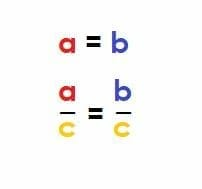#### Converse of the Division Property of Equality The converse of the division property of equality is also true. That is, let$a, b, c$be real numbers such that$a\neq b$and$c\neq0$. Then$\frac{a}{c}\neq \frac{b}{c}$. Put another way, let$a, b, c,$and$d$be real numbers such that$a=b$,$c\neq0$, and$d\neq0$. Then$\frac{a}{c}= \frac{b}{d}$, then$c=d$. ### Uses for the Division Property of Equality Like the other similar properties of equality, the division property of equality has uses in both arithmetic and algebra. In arithmetic, the division property of equality helps to decide whether two math terms are equal. In algebra, the division property of equality justifies steps when solving for an unknown value. Doing this requires getting a variable by itself. Division will undo any multiplication done to a variable. ### Is the Division Property of Equality an Axiom? The division property of equality derives from the multiplication property of equality. Thus, axiom lists do not need to have it. However, most lists of them do. Euclid did not define the division property of equality or the multiplication property of equality in his Elements. This is notable since he did define several others. The most likely reason for this is that neither property has many uses in the planar geometry he was working on. Giuseppe Peano made his list of arithmetic axioms in the 1800s. He did not directly include the division property of equality. This list was meant to make sure mathematical rigor when logic-based math was taking off. However, his axioms are usually augmented with addition and multiplication. Division follows from these. Thus, even though the division property of equality is deducible from other axioms, it is often listed as an axiom in its own right. It has a lot of uses, so this makes reference easy. Note, however, that is is possible to deduce the multiplication property of equality from the division property of equality. Example 3 does just that. ### Division Property of Equality Example Like the multiplication property of equality, Euclid did not define the division property of equality in his Elements. As a result, there are not any famous geometric proofs that rely on it. There is a famous example of the necessity of the statement that$c\neq0$though. Skipping this requirement can lead to logical errors. This is shown in the example below. Let$a$and$b$be real numbers such that$a=b$. Then: 1.$a^2=ab$by the multiplication property. 2.$a^2-^2=ab-b^2$by the subtraction property. 3.$(a+b)(a-b)=b(a-b)$by the distributive property. 4.$(a+b)=b$by the division property. 5.$2b=b$by the substitution property. 6.$2=1$by the division property.$2\neq1$. Clearly, there is some mistake in this logic. The problem was in step 4. Here,$a-b$divides both sides. But, since$a=b$, the substitution property states that$a-b=a-a=0$. Dividing by$0$in step 4 was the logical flaw. ### Examples This section covers common examples of problems involving division property of equality and their step-by-step solutions. ### Example 1 Let$a, b, c,$and$d$be real numbers such that$a=b$and$c=d$. Assume$a\neq0$and$c\neq0$. Use the division property of equality to determine which of the following are equivalent. •$\frac{a}{c}$and$\frac{b}{c}$•$\frac{a}{c+d}$and$\frac{b}{c+d}$•$\frac{a}{c-d}$and$\frac{b}{c-d}$### Solution The first two pairs are equivalent, but the third pair is not. Recall that$c$is not equal to$0$and$a$is equal to$b$. The division property of equality says that$\frac{a}{c}$and$\frac{b}{c}$must be equal.$c\neq0$, but$c$is equal to$d$. If$c+d=0$, the substitution property of equality states that$c+c$is also equal to$0$. This simplifies to$2c=0$. The multiplication property then states that$c=0$. Therefore, since$c \neq0$,$c+d$is not equal to$0$either. Therefore, according to the division property of equality,$\frac{a}{c+d}$and$\frac{b}{c+d}$. However, since$c=d$, the substitution property of equality says that$c-d=c-c$. Since$c-c=0$,$c-d=0$by the transitive property. Thus, dividing by$c-d$is the same as dividing by$0$. Therefore, equality does not hold and$\frac{a}{c-d}$and$\frac{b}{c-d}$are not equal. ### Example 2 Two small local libraries have the same number of books. Each library divides its books evenly among 20 shelves. How do the number of books on each shelf at the first small library compare to the number of books on the each shelf at the second small library. ### Solution Let$f$be the number of books at the first library and let$s$be the number of books at the second library. It is given that$f=s$. The first library divides all of its books evenly among 20 shelves. This means that each shelf has$\frac{f}{20}$books. The second one also divides all of its books evenly among 20 shelves. This means that each shelf has$\frac{s}{20}$books. Note that$20\neq0$. Thus, the division property of equality states that$\frac{f}{20}=\frac{s}{20}$. In other words, the number of books on each shelf is the same at both places by the division property of equality. ### Example 3 Prove the division property of equality using the multiplication property of equality. ### Solution Recall the multiplication property of equality. It states that if$a, b,$and$c$are real numbers such that$a=b$, then$ac=bc$. Using the division property of equality to prove this means first assuming the division property of equality is true. That is, assume$a, b$are real numbers such that$a=b$and$c\neq0$. Then$\frac{a}{c}=\frac{b}{c}$. Note that is$c\neq0$, then$\frac{1}{c}$is a real number. Thus,$\frac{a}{\frac{1}{c}}=\frac{b}{\frac{1}{c}}$. This simplifies to$a\times c=b\times c$or$ac=bc$. Thus, if$a, b,$and$c$are real numbers such that$a=b$and$c\neq0$, then$ac=bc$. In other words, the multiplication property of equality holds for any real number$c\neq0$. But the multiplication property of equality holds for any real number$c$. Therefore, it is required to prove that$a\times0=b\times0$. Since any number times$0$is$0$,$a\times0=0$and$b\times0=0$. Therefore, the transitive property of equality states that$a\times0=b\times0$. Thus, if the division property of equality is true, the multiplication property of equality is true. ### Example 4 Let$x$be a real number such that$5x=35$. Use the division property of equality to prove that$x=7$. ### Solution It is required to get the variable by itself to solve for$x$.$x$is multiplied by$5$. This means dividing by$5$will do just that. The division property of equality states that doing this to both sides keeps the equality. Thus,$\frac{5x}{5}=\frac{35}{5}$. This simplifies to:$x=7$Thus, the value of$x$is$7$. ### Example 5 Let$x$be a real number such that$4x=60$. Let$y$be a real number such that$6x=90$. Prove that$x=y$. Use the division property of equality and the transitive property of equality to do it. ### Solution First, solve for both$x$and$y$.$x$is multiplied by$4$. Thus, isolate the variable by dividing by$4$. However, to keep the equality, the division property of equality requires doing this to both sides. Thus,$\frac{4x}{4}=\frac{60}{4}$. This becomesto$x=15$.$y$is multiplied by$6$. Thus, isolate the variable by dividing by$6$. However, to keep equality, the division property of equality requires also doing this to both sides. Thus,$\frac{6x}{6}=\frac{90}{6}$. This simplifies to$y=6$. Now$x=6$and$y=6$. The transitive property of equality states that$x=y$, as required. ### Practice Problems 1. Let$a, b, c, d$be real numbers such that$a=b$and$c=d$. Let$a\neq0$and$c\neq0$. Use the division property of equality to determine which of the following pairs are equivalent. A.$\frac{a}{cd}$and$\frac{b}{cd}$B.$\frac{a}{\frac{1}{c+d}}$and$\frac{b}{\frac{1}{c+d}}$C.$\frac{a}{c}$and$\frac{b}{d}
2. Two summer camps have the same number of campers. Each summer camp wants to ensure that they have a low camper to counselor ratio. The first summer camp has $8$. The second summer camp also has $8$ counselors. How does the ratio of campers per counselor compare at the two summer camps?
3. Prove that the number $1$ is the multiplicative identity using the division property of equality. That is, prove that if $a$ and $c$ are real numbers such that $ac=a$, then $c=1$.
4. Let $x$ be a real number such that $\frac{4x}{5}=32$. Use the division property of equality to prove $x=40$.
5. Let $a, b, c, d,$ and $x$ be real numbers and let  such that $\frac{abx}{5c}=\frac{2ac+d}{b-1}.$ Assume $5c\neq0$ and $b-1\neq0$. Solve for $x$ using the division property of equality.

1. All three are equivalent. Since $c\neq0$, $cd=c^2\neq0$. Therefore, A is equal. Likewise, $c+d=c+c=2c\neq0$. Therefore, B is equal. Finally, by the substitution property of equality, $\frac{b}{d}=\frac{b}{c}$.
2. The ratio will be the same by the division property of equality.
3. Let $a, b,$ and $d$ be real numbers such that $a=b$ and $d\neq0$. Then $\frac{a}{d}=\frac{b}{d}$.
Consider the multiplicative identity $c$ such that $ac=a$ for any real number $a$. Then, as long as $a\neq0$, $\frac{ac}{a}=\frac{a}{a}$.
This simplifies to $c=1$. Therefore, $1$ is the multiplicative identity. QED.
4. Note that $\frac{4x}{5}=\frac{4}{5}x$. The division property of equality states that dividing both sides by $\frac{4}{5}$ keeps equality. This, however, is the same as multiplying both sides by $\frac{5}{4}$. This is $\frac{5}{4}\times\frac{4}{5}x=\frac{5}{4}\times32$. Simplifying yields $x=40$. Thus, $x$ is equal to $40$ as required. QED.
5. $\frac{abx}{5c}=\frac{ab}{5c}x$. Therefore, dividing both sides by $\frac{ab}{5c}$ keeps the equality. But, dividing by $\frac{ab}{5c}$ is the same as multiplying by $\frac{5c}{ab}$. Therefore, $\frac{5c}{ab}\times\frac{ab}{5c}x = \frac{5c}{ab}\times\frac{2ac+d}{b-1}$. This simplifies to $x = \frac{(5c)(2ac+d)}{(ab)(b-1)}$.
Sours: https://www.storyofmathematics.com/division-property-of-equality
Division Property of Equality

## Division Property Of Equality — Definition & Example

### Division property of equality definition

The Division Property of Equality says that dividing both sides of an equation by the same number does not affect the equation. Another way to consider it is that if you divide one side of an equation by a number, you must divide the other side by the same number.Multiplication and division share similar properties and effects on equations.

### Division property of equality formula

The Division Property of Equality states:

This is very similar to the multiplication property of equality, in which we can multiply both sides of any equation without affecting the equation.The properties of equality are:

• Division property of equality
• Subtraction property of equality
• Multiplication property of equality

### Division Property Of Equality Example

Division is a method of sharing fairly. In equations, you need to operate on both sides of the equals sign fairly. Whatever you do to the left side of the equal sign, you must do it to the right side of the equation as well.

You cannot take away or divide something from one side without doing the same thing to the other side.

Suppose you are sharing pans of donated brownies among all the members of your Math Club.

You need to serve from both pans equally, so the donors will not have their feelings hurt. You dish out brownies from the first pan, so you dish out brownies from the second pan, too.

Division is really just fast, repeated subtraction, so instead of just dishing out brownies from each pan, we can cut both pans into brownies and divide them into piles of :

We had brownies on both sides, each divided by . Both sides of our brownie equation show piles of brownies in each pile.

The Division Property of Equality works with all real numbers and with algebraic expressions using variables.

Here are examples using integers:Here is an example with fractions:

Here is an example using mixed numbers:

Here is an example using decimals:

Here is an example using variables:### What you learned:

After working your way through this lesson and video, you have learned:

• The definition of the division property of equality
• The division property of equality formula
• How to use the division property of equality for integers, fractions, mixed numbers, decimals, and irrational numbers
• Examples of the division property of equalityInstructor: Malcolm M.
Malcolm has a Master's Degree in education and holds four teaching certificates. He has been a public school teacher for 27 years, including 15 years as a mathematics teacher.

Sours: https://tutors.com/lesson/division-property-of-equality

## Division Property Of Equality - Definition with Examples

### What is Division Property of Equality?

At times we refer to algebra as generalized arithmetic. Algebraic thinking plants seeds for many higher-order concepts as well helps in understanding the other domains of science.

The equation is a mathematical sentence with an equal sign, and it is one of the essential elements Algebra.

ExampleThe operations of addition, subtraction, multiplication and division do not change the truth value of any equation.

The division property of equality states that when we divide both sides of an equation by the same non-zero number, the two sides remain equal.

That is, if a, b, and c are real numbers such that a = b and c ≠0, then a c =a c .

Example: Consider the equation 12 = 12.

Divide both sides by 4.

12 4 =12 4

3 = 3

That is, the equation still remains true.

Note, that the divisor cannot be zero as the division by zero is not defined.

This property is used in solving equations.

Example: 6x = 24

Divide both sides by 6.

6x 6 =24x 6

6x6=246

x=4

To check we can substitute the value of x in the original equation.

6×4=24

24 = 24

Example: Rhea bought 7 notebooks for $21. What is the cost of each notebook? Let a be the cost of each notebook. Then, 7 times a is the total cost,$21.

That is, 7a = 21.

By the division property of equality, if you divide both sides by the same non-zero number, the equality still holds true. So, divide both sides by 7.

17a 7 =21 7

a=3

Therefore, the cost of each notebook is \$3.

 Fun Fact:Another form of the property is if a, b, c, and d are real numbers such that a = b, c = d and c, d ≠0, then a c =b d .
Sours: https://www.splashlearn.com/math-vocabulary/division/division-property-of-equality
Multiplication and Division Property of Equality

She lay on her back, we crawled up to her and put our members to her lips, she took mine in one hand. And a friend in the other and began to drive her tongue over the head of one or the other with her tongue. Then she began to suck a friend's penis, and my only licked. Then a friend climbed on top of her and began to fuck her, and she jerked off me.

He pulled out his penis from her vagina and finished on her, and I ended up with him at the same time on her, when she saw.

### You will also be interested:

I see how the nipples of my victim are gradually filling with excitement, hardening. - Sit still, otherwise everything will end without beginning, my sweet. - Yes, master. I bring a bag, in it I have brought things, everything for what I have in mind. I take out a set of needles for piercing, a white gold ring, which was made according to my order, a means for treating the.

1739 1740 1741 1742 1743